# 2021年成人高考高起点《理数》模拟试题三

2021-03-03 15:46 江西成考网

1.在等差数列{an}中，已知a1+a2+a3+a4+a5=15,那a3=

A.3
B.4
C.5
D.6

2.A.大于0
B.小于0
C.等于0
D.大于0或等于0

3.A.充分但非必要条件
B.必要但非充分条件
C.充要条件
D.非充分非必要条件

4.A.大于0
B.小于0
C.等于0
D.大于0或等于0

5.已知a+b>0，b<a，那么下列关系正确的是

A.a>6>-a>-b
B.a>-a>b>-b
C.a>-b>b>-a
D.-a>-b>a>b

6.用0，1，2，…，9这十个数字，可以组成没有重复数字的三位数的个数为

A.720
B.648
C.620
D.548

7.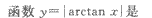A.增函数
B.减函数
C.x>0时是增函数，x<0时是减函数
D.x=>0时是减函数，x<0时是增函数

8.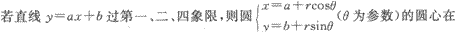A.第一象限
B.第二象限
C.第三象限
D.第四象限

9.A.A
B.B
C.C.
D.D

10.|a|=10,b=(-3,4)且口与b同方向，则a=

A.(6,8)
B.(6,-8)
C.(-6,8)
D.(3,4)

11.已知函数f(x)=a2+k的图象经过点(1，7)，且其反函数f-1(x)的图像经过点(4，0)，则函数f(x)的表达式是 （　　）

A.f(x)=4x+3
B.f(x)=2x+5
C.f(x)=5x+2
D.f(x)=3x+5

12.A.奇函数
B.偶函数
C.非奇非偶函数
D.既奇又偶函数

13.下列函数为偶函数的是

A.y=-x
B.y=x sinx
C.y=x cosx
D.y=x2+x

14.A.1
B.-1
C.0
D.i

15.两条直线垂直于同一条直线，这两条直线的关系为（　　）

A.平行
B.相交
C.异面
D.位置不确定

16.若关于x的二次方程a(1+x2)+2bx=c(1-x2)有两个相等实根，则以正数a，b，c为边长的三角形是 （　　）

A.锐角三角形
B.钝角三角形
C.直角三角形
D.任意三角形

17.任选一个小于10的正整数，它不是素数的概率为

A.B.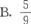C.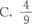D.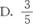18.19. 抛物线x2=-2py(p>0)上各点与直线3x+4y-8=0的最短距离为1，则__________

20.给定三点A(1，0)、B(-1，O)、C(1，2)，那么通过点A，并且与直线BC垂直的直线方程是__________．

21.当x∈__________时，函数y=2x3-6x2+7为减函数．

22.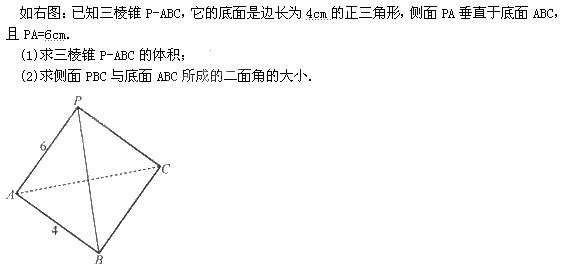23.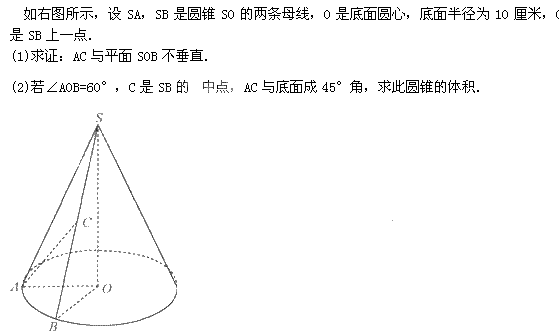24.25.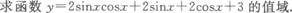437
2021年成人高考考试时间
10月23,24日+